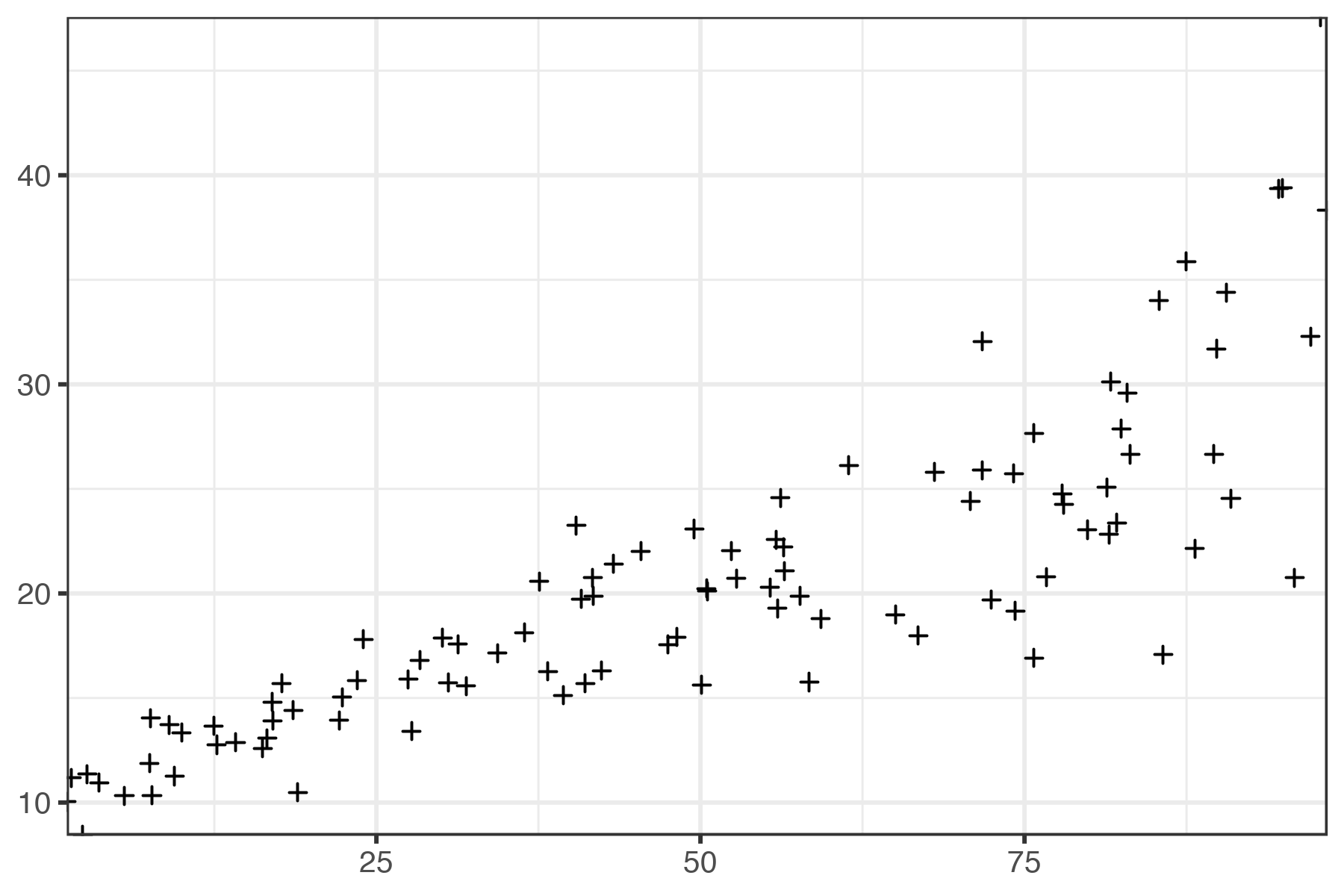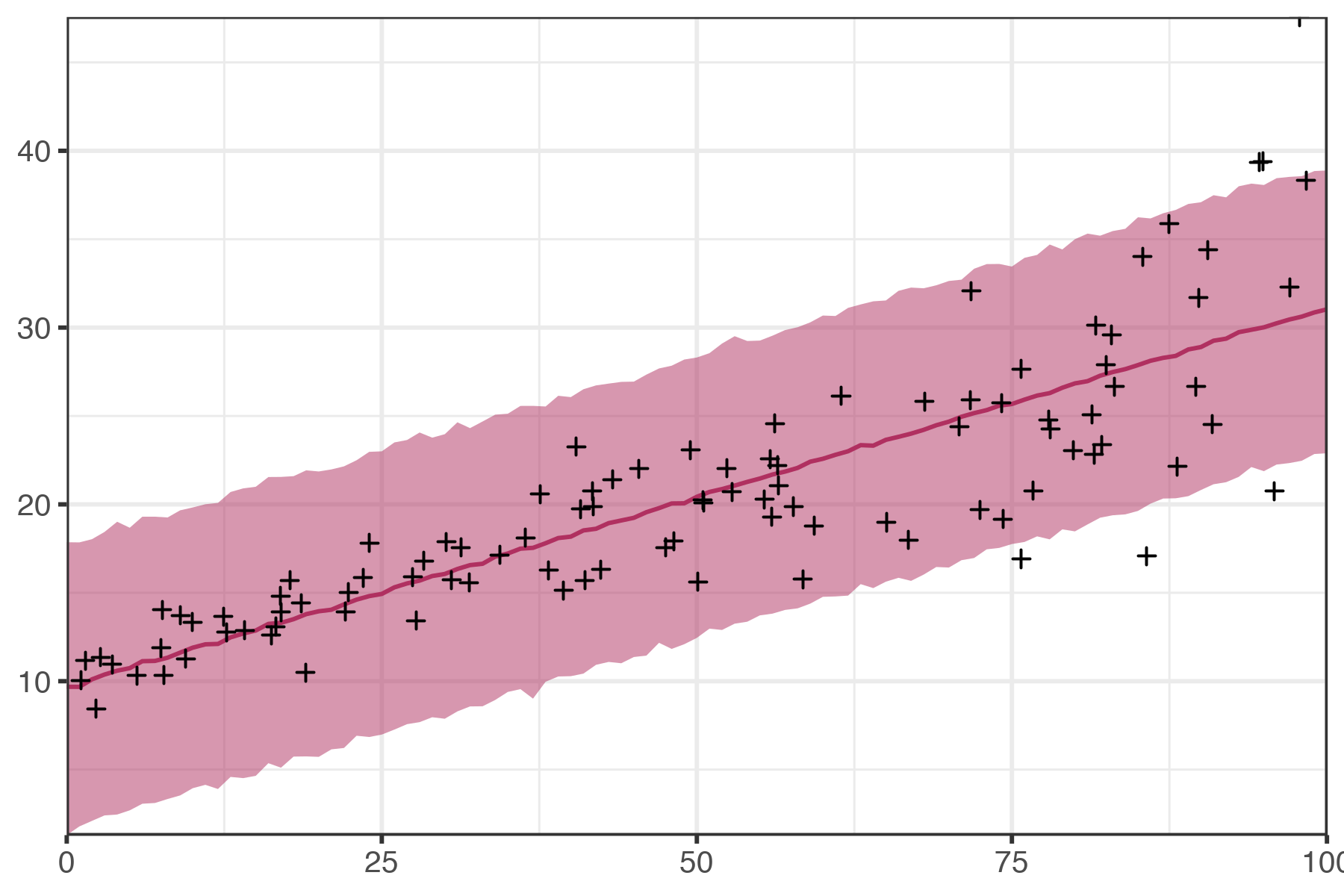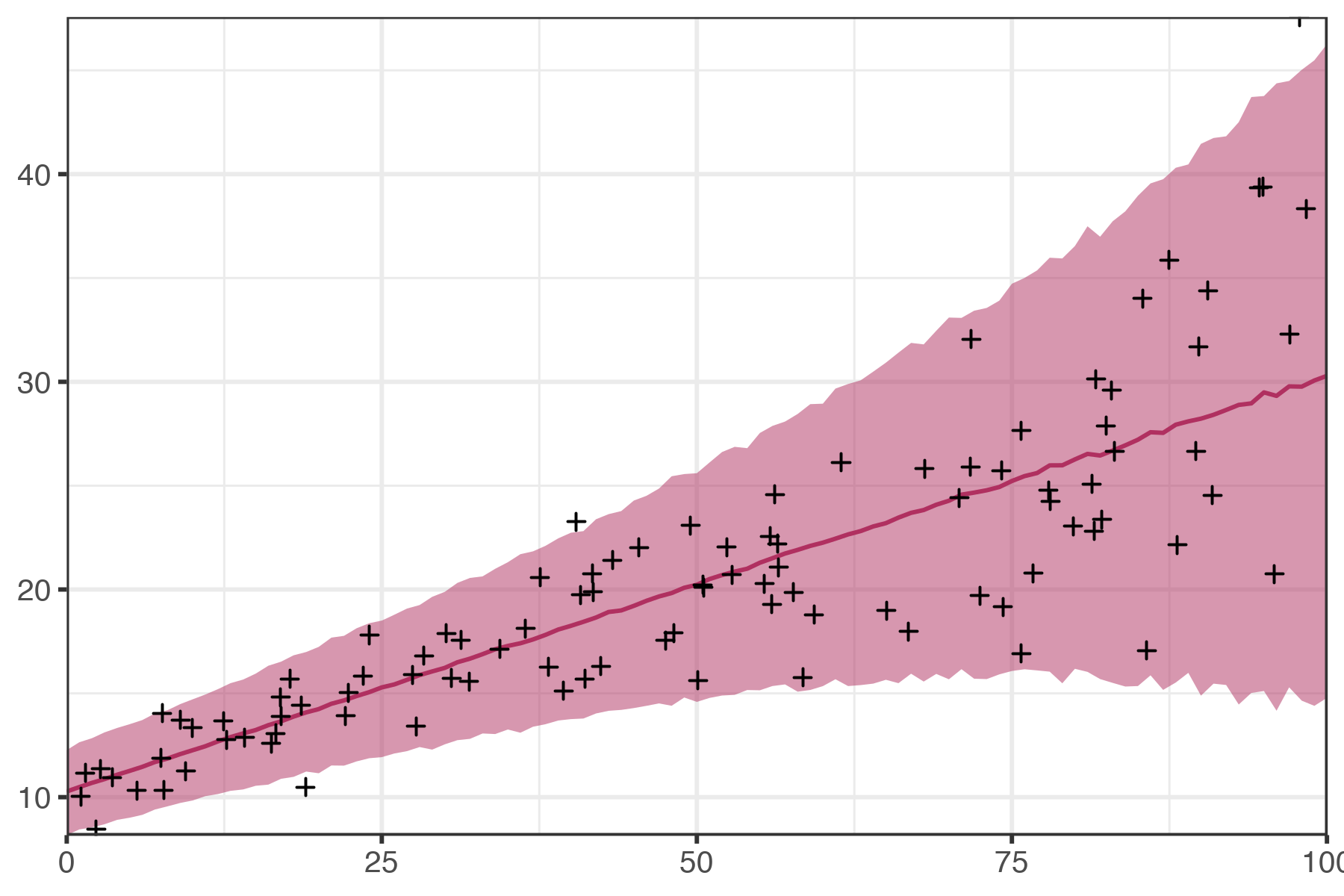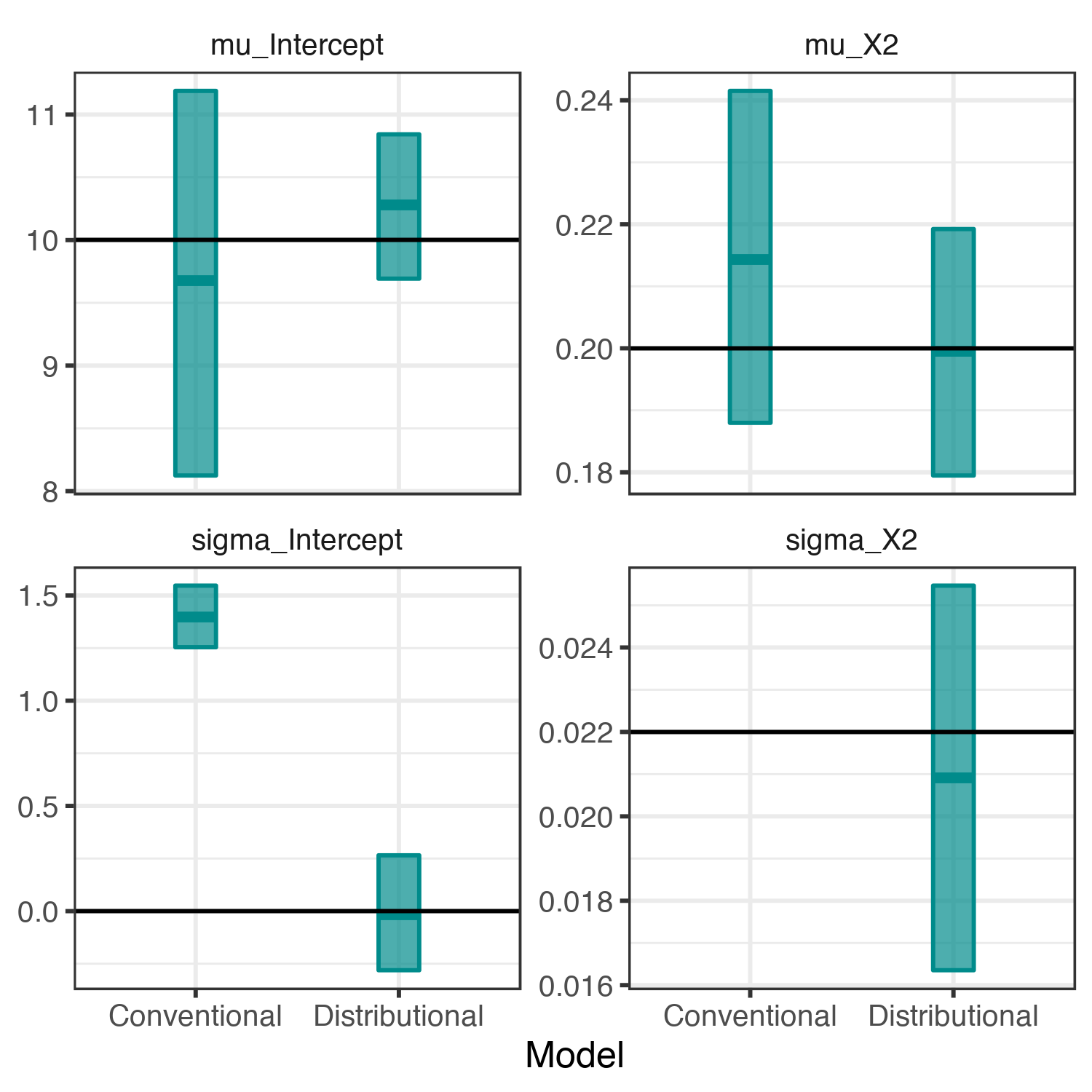## Fitting Distributional Regression Models in brms: a Brief Tutorial

“Someone must have done this before,” I mutter to myself as I type “what do you call it when…” into Google. I am convinced that one of the hardest parts of doing research is knowing what terms to search for. Modern search engines handle incoherent queries like “binomial distribution but with weights please” surprisingly well, but it can only do so much when I truly don't know what something is called.

I ran into a surprising instance of this problem a few months ago when I fell into a project modeling sexual partner age distributions. People select partners in fairly predictable ways, and the resulting age distributions exhibit clear patterns with respect to age and sex. The stumbling block for this project was that the whole distributions seemed to vary across the dataset. For example, middle aged people's partners have much more variable ages than teenagers' partners. In fact, we observed systematic variation by age and sex in each of the first four moments of the data; men aged 34 years exhibited systematically different kurtosis than women aged 23 years.

The obvious thing to do was to model each of those moments as a function of data (not just the mean), but no one seemed to know what that would be called (or if it was even legal). Many Google searches of the form “what do you call it when you model the variance too?” later, I found this vignette from the brms R package by Dr. Paul Bürkner. Dr. Bürkner identified what I was looking for as “distributional regression” and questioned why it wasn't more widely used. Someone had indeed done it before and had already implemented it in well-regarded statistical inference framework. If only it worked out so well every time.

That vignette is an extremely useful resource, but I wasn't able to find a worked, introductory example to the topic. At that point, I wanted to make sure I understood the basics of the method and its implementation. In this post, I will provide an overview of the justification for and mechanics of distributional regression methods, as well as an detailed demonstration of how to fit a distributional regression model to heteroscedastic data in brms.

## What is Distributional Regression?

Distributional regression arises naturally when we look closely at the assumptions made by conventional Bayesian regression. In the Gaussian case of Bayesian regression, we assume that each observation of a response variable, $$y_i$$, is normally distributed around an inferred location and standard deviation:

$y_i \sim \mathrm{N}(\mu_i, \sigma)$

We typically assume that $$\mu_i$$ is some linear transformation of data, $$X_i$$, and that $$\sigma$$ is constant across all observations. We all know that assuming that $$\mu_i$$ is a linear transformation of data is only a convenient-but-inaccurate approximation in most cases, but I would argue that not allowing $$\sigma$$ to vary with respect to data could actually be the less realistic of the two assumptions. Why should we expect that every single observation's generating process has exactly the same variance?

Instead, we can allow $$\sigma$$ to vary with respect to data as well, leading to the following linear distributional model:

$\begin{array}{rcl} y_i &\sim&\mathrm{N}(\mu_i, \sigma_i) \newline \mu_i &=&\beta^\mu X_i ^\mu \newline \log\sigma_i &=&\beta^\sigma X_i ^\sigma \end{array}$

Note that we do not require $$X^\mu = X^\sigma$$. In practice, we have found that even this small change can allow models to explain far more variation than would be possible under conventional regression.

To see this in practice, we will simulate a dataset that might appear to adequately modeled by conventional regression, but actually requires distributional regression.

## Fake Data Simulation

First, we need to generate data that might benefit from distributional regression. The case that everyone learned about in their undergrad Statistics course are data that exhibit heteroscedasticity. We call a collection of random variables “heteroscedastic” when their variability varies along some (hopefully) measurable dimension. In this case, we will simulate a relatively small dataset (100 observations) to test distributional regression in a case where we might not consider it a possibility.

Generating heteroscedastic data is straightforward enough in R. Step zero is to fix a seed and load in the packages we will need for the analysis.

library(ggplot2)
library(brms)
set.seed(404)


Then, we generate a matrix, X, with 100 rows and 2 columns (one intercept and one covariate) that we will use to generate each observation's true mean and standard deviation. We fix the coefficients for the mean and standard deviation, B_mu and B_sigma, respectively, and find the true means and standard deviations, mu and sigma. Finally, we take one normally distributed sample per observation according to mu and sigma. We use a log-linear model for sigma to ensure that it will always be positive.

N <- 100
X <- cbind(1, runif(N, 0, 100))

B_mu <- c(10, 0.2)
B_sigma <- c(0, 0.022)

mu <- X %*% B_mu
sigma <- exp(X %*% B_sigma)

y <- rnorm(N, mu, sigma)


We can use ggplot2 to check that our fake data simulation works as expected, noting that we see the expected “fan” shape.

ggplot() +
geom_point(aes(x = X[,2], y = y), shape=3) +
scale_x_continuous(expand=c(0,0)) +
scale_y_continuous(expand=c(0,0)) +
labs(y=NULL, x = NULL)## Conventional Regression

If we were less careful, we would proceed with plain old Bayesian linear regression, in which we have (omitting priors):

$\begin{array}{rcl} y_i &\sim&\mathrm{N}(\mu_i, \sigma) \newline \mu_i &=& \beta X_i. \end{array}$

In other words, we have a linear model for each observation's mean, but assume that $$\sigma$$ is constant across the entire dataset. Because we have generated our data from scratch, we know for a fact that this assumption is wrong.

We can implement this model quickly with brms. First, we put our data into a convenient data.frame. Then we define our “conventional” regression model using typical R formula syntax. Note that brms will automatically add a constant if we do not specify … + 0 or … - 1. Finally, we fit the model using the brm function. I am suppressing the output from this step to hide some compiler warnings.

sim_data <- data.frame(y, X)

conv_fm <- bf(
y ~ X2
)
conv_brm <- brm(conv_fm, data = sim_data)


Here is the output from that model:

print(conv_brm)

##  Family: gaussian
##   Links: mu = identity; sigma = identity
## Formula: y ~ X2
##    Data: sim_data (Number of observations: 100)
## Samples: 4 chains, each with iter = 2000; warmup = 1000; thin = 1;
##          total post-warmup samples = 4000
##
## Population-Level Effects:
##           Estimate Est.Error l-95% CI u-95% CI Rhat Bulk_ESS Tail_ESS
## Intercept     9.68      0.79     8.12    11.19 1.00     4042     3034
## X2            0.21      0.01     0.19     0.24 1.00     4089     3161
##
## Family Specific Parameters:
##       Estimate Est.Error l-95% CI u-95% CI Rhat Bulk_ESS Tail_ESS
## sigma     4.05      0.30     3.50     4.70 1.00     3676     2782
##
## Samples were drawn using sampling(NUTS). For each parameter, Bulk_ESS
## and Tail_ESS are effective sample size measures, and Rhat is the potential
## scale reduction factor on split chains (at convergence, Rhat = 1).


First, we note that we have fit a “gaussian” regression, which has two parameters: mu and sigma. All of the Rhat values are 1.00, so everything appears to be in order. In general, the coefficient estimates are not too far from the truth.

Most importantly, if we didn't know for a fact that this model was wrong, we would look at our (very precise) estimates and assume that everything was working well. Fortunately, we can take advantage of the posterior checking tools brms provides to see how wrong the model is. In particular, we can find the posterior predictive distribution at regularly spaced values of X2. Here, the predict function is actually taking samples from the posterior distribution, not just returning the predicted mean.

pred_df <- data.frame(X2 = seq(0, 100))

conv_post_pred <- cbind(pred_df, predict(conv_brm, newdata = pred_df))
head(conv_post_pred)

##   X2  Estimate Est.Error     Q2.5    Q97.5
## 1  0  9.683700  4.177371 1.292286 17.85911
## 2  1  9.678432  4.143473 1.783049 17.84957
## 3  2 10.103117  4.054727 2.096661 18.03102
## 4  3 10.375159  4.095678 2.407267 18.44198
## 5  4 10.592883  4.198666 2.457239 19.01788
## 6  5 10.737160  4.124102 2.693994 18.67832


We can plot the predicted intervals with our data to see how our model performs:We would like the red region to cover 95% of the data points and generally replicate the patterns we observed, which it seems to do. The heteroscedasticity built into the dataset is very hard to see from this perspective (try covering half the image with your hand). Conventional regression is operating exactly as expected, which, in this case, means that we are making an explicit (and incorrect) assumption that the variance of the outcome variable is constant across the whole dataset.

## Distributional Regression

Instead, we can move into a distributional regression framework:

$\begin{array}{rcl} y_i &\sim&\mathrm{N}(\mu_i, \sigma_i) \newline \mu_i &=& \beta^\mu X^\mu_i \newline \log\sigma_i &=&\beta^\sigma X^\sigma_i. \end{array}$

Note that $$\sigma$$ is now indexed by observation and that we have introduced parameter-specific coefficients and design matrices. We do not need to assume that $$X^\mu$$ and $$X^\sigma$$ are identical, but in this demonstration, they are.

We can define slightly more complex bf object to fit this model. The first formula implicitly corresponds to the mu parameter, which is required by all brms families, and must have the desired outcome variable as its dependent variable. Each subsequent formula can correspond to any of the other (non-mu) distributional parameters in the family. We have already noted from our first model that the “gaussian” family expects mu and sigma, so our second formula has sigma as its dependent variable.

dist_fm <- bf(
y ~ X2,
sigma ~ X2
)
dist_brm <- brm(dist_fm, data = sim_data)


This model takes just a bit longer to fit that the previous one and returns the following results

print(dist_brm)

##  Family: gaussian
##   Links: mu = identity; sigma = log
## Formula: y ~ X2
##          sigma ~ X2
##    Data: sim_data (Number of observations: 100)
## Samples: 4 chains, each with iter = 2000; warmup = 1000; thin = 1;
##          total post-warmup samples = 4000
##
## Population-Level Effects:
##                 Estimate Est.Error l-95% CI u-95% CI Rhat Bulk_ESS Tail_ESS
## Intercept          10.28      0.30     9.69    10.84 1.00     3298     3131
## sigma_Intercept    -0.02      0.14    -0.28     0.27 1.00     2643     2178
## X2                  0.20      0.01     0.18     0.22 1.00     1965     2268
## sigma_X2            0.02      0.00     0.02     0.03 1.00     3250     2540
##
## Samples were drawn using sampling(NUTS). For each parameter, Bulk_ESS
## and Tail_ESS are effective sample size measures, and Rhat is the potential
## scale reduction factor on split chains (at convergence, Rhat = 1).


Here, Intercept and X2 have an implicit mu_ in front of them, and sigma_Intercept and sigma_X2 comprise $$\beta^\sigma$$. Again, we see that each Rhat value is 1.00, suggesting that the four chains have mixed well. We also note that sigma now has a log link function, which we have slyly anticipated in our fake data simulation.

We can find and plot posterior predictions as before.

dist_post_pred <- cbind(pred_df, predict(dist_brm, newdata = pred_df))
head(dist_post_pred)

##   X2 Estimate Est.Error     Q2.5    Q97.5
## 1  0 10.27464  1.042657 8.173381 12.26846
## 2  1 10.48839  1.068219 8.446713 12.64576
## 3  2 10.68979  1.086983 8.547180 12.83999
## 4  3 10.87010  1.112608 8.697068 13.12031
## 5  4 11.08150  1.119850 8.908548 13.33388
## 6  5 11.26817  1.126003 9.012126 13.51619Unsurprisingly, the correct model appears to fit far better than the incorrect model, but we cannot say for sure that the extra complexity is valuable without more robust model comparisons.

## Model Comparisons

The first question we can ask is: did each model recover the true values? We can extract the estimated coefficients and intercepts and plot them against the true values to see.

conv_fixef <- data.frame(fixef(conv_brm))
conv_fixef$variable <- rownames(fixef(conv_brm)) tmp_sigma <- data.frame(log(t(summary(conv_brm)$spec_pars[1:4])),variable = 'sigma_Intercept')
names(tmp_sigma) <- names(conv_fixef)
conv_fixef <- rbind(conv_fixef, tmp_sigma)
conv_fixef$Model <- 'Conventional' dist_fixef <- data.frame(fixef(dist_brm)) dist_fixef$variable <- rownames(fixef(dist_brm))
dist_fixef$Model <- 'Distributional' true_fixef <- data.frame( variable = c('mu_Intercept', 'mu_X2', 'sigma_Intercept', 'sigma_X2'), value = c(B_mu, B_sigma) ) all_fixef <- rbind(conv_fixef, dist_fixef) all_fixef$variable[all_fixef$variable == 'Intercept'] <- 'mu_Intercept' all_fixef$variable[all_fixef$variable == 'X2'] <- 'mu_X2' ggplot() + geom_crossbar(data = all_fixef, aes(x=Model, y = Estimate, ymin = Q2.5, ymax=Q97.5), width=0.2, fill='darkcyan', color='darkcyan', alpha=0.7) + geom_hline(data = true_fixef, aes(yintercept=value)) + facet_wrap('variable', scales='free_y') + labs(y = NULL)Here, each crossbar is the point estimate and 95% credible interval, and the horizontal lines are the true values. We can see that both models actually do relatively well inferring the true parameters for mu. However, the sigma_Intercept panel illustrates how inappropriate our estimate of $$\log\sigma$$ is when X2 is equal to zero. We can also estimate and compare the two models' expected log posterior densities (ELPDs) to estimate which model would offer better out-of-sample fit. With the loo function built-in to brms (borrowed from the loo package), we can easily estimate and compare these ELPDs. Here, loo_res will contain the ELPD estimates for both models, as well as the differences between the two models. Higher ELPD is better. loo_res <- loo(conv_brm, dist_brm) loo_comparison <- loo_res$diffs

print(loo_res)

## Output of model 'conv_brm':
##
## Computed from 4000 by 100 log-likelihood matrix
##
##          Estimate   SE
## elpd_loo   -283.9 12.2
## p_loo         5.2  2.3
## looic       567.8 24.4
## ------
## Monte Carlo SE of elpd_loo is 0.1.
##
## Pareto k diagnostic values:
##                          Count Pct.    Min. n_eff
## (-Inf, 0.5]   (good)     99    99.0%   1781
##  (0.5, 0.7]   (ok)        1     1.0%   218
##    (0.7, 1]   (bad)       0     0.0%   <NA>
##    (1, Inf)   (very bad)  0     0.0%   <NA>
##
## All Pareto k estimates are ok (k < 0.7).
## See help('pareto-k-diagnostic') for details.
##
## Output of model 'dist_brm':
##
## Computed from 4000 by 100 log-likelihood matrix
##
##          Estimate   SE
## elpd_loo   -246.9  9.1
## p_loo         4.1  1.0
## looic       493.7 18.2
## ------
## Monte Carlo SE of elpd_loo is 0.1.
##
## Pareto k diagnostic values:
##                          Count Pct.    Min. n_eff
## (-Inf, 0.5]   (good)     98    98.0%   1563
##  (0.5, 0.7]   (ok)        2     2.0%   594
##    (0.7, 1]   (bad)       0     0.0%   <NA>
##    (1, Inf)   (very bad)  0     0.0%   <NA>
##
## All Pareto k estimates are ok (k < 0.7).
## See help('pareto-k-diagnostic') for details.
##
## Model comparisons:
##          elpd_diff se_diff
## dist_brm   0.0       0.0
## conv_brm -37.0       8.6


Interestingly, the estimated number of parameters is slightly lower in the distributional model. The absolute value of the ratio of the difference to the standard error in the difference is 4.3, suggesting that the distributional model is significantly better than the conventional model. Again, given that the second model is literally correct, this just confirms that the inference machine is functioning as expected.

## So what?

The distributional model we built in this post doesn't even begin to scratch the surface of what we could do with this framework. Building models in brms gives us access to a wide set of sophisticated prior distributions, regression families, and hierarchical modeling tools, any of which could be applied to any distributional parameter. For example, if our data were collected at heterogeneous sites, we could use a hierarchical model to allow each site to have a completely distinct distribution.

In the original application (modeling sexual partner age distributions), I integrated these techniques with another fabulous feature from brms: custom families. We were able to model the first four moments of these distributions as functions of respondent age and sexm not just the first. These models replicated observed partner age distributions far more accurately than previously possible.

Hopefully, this tutorial offered enough context to help you get started using distributional models in brms. The formula syntax and built-in model comparison tools make it easy to fit distributional models alongside conventional regression models. We will not always have enough data to fit complex distributional models, but, if nothing else, we do always need to think carefully about the level at which our models assume homogeneity.